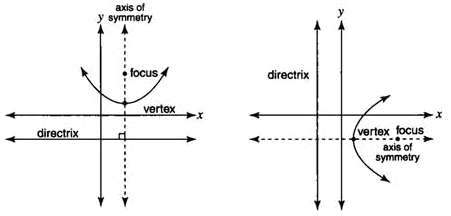Parabolas

Parabolas were previously presented in function form. This book will introduce the conic section applications of parabolas. The connection to conic sections will not change the characteristics of parabolas. However some may seem new.

A parabola is the set of points in a plane that are the same distance from a given point and a given line in that plane. The given point is called the focus, and the line is called the directrix. The midpoint of the perpendicular segment from the focus to the directrix is called the vertex of the parabola. The line that passes through the vertex and focus is called the axis of symmetry.